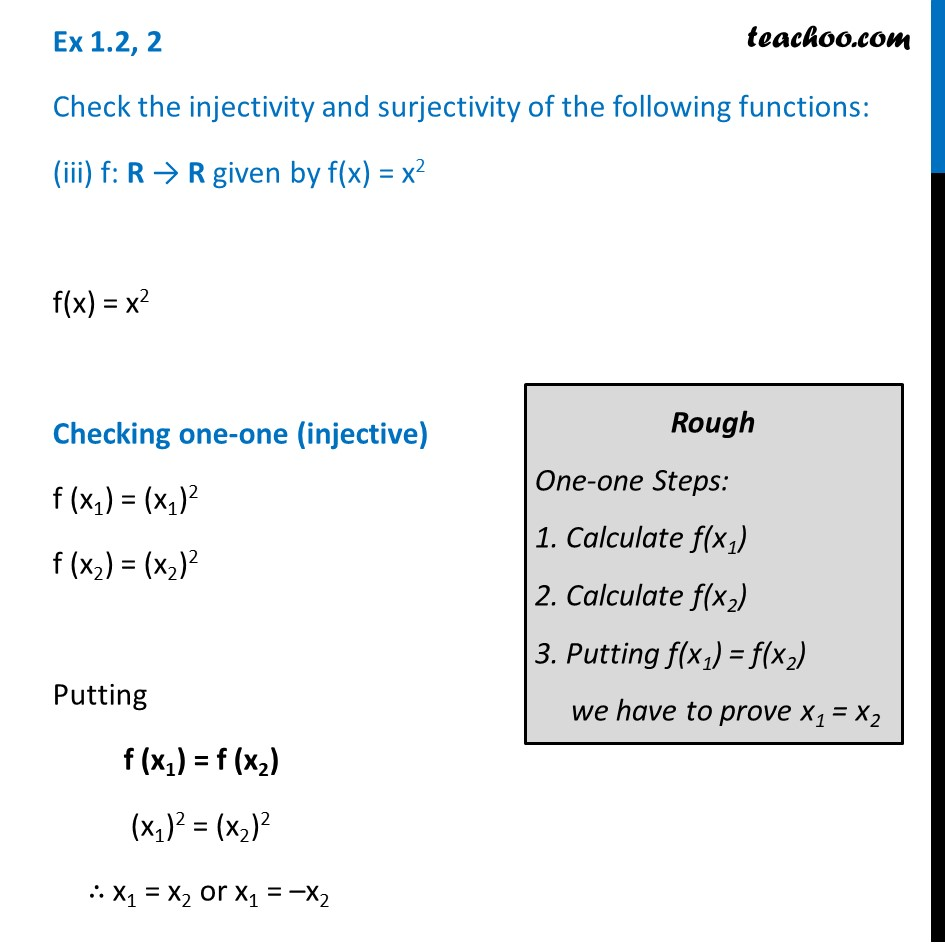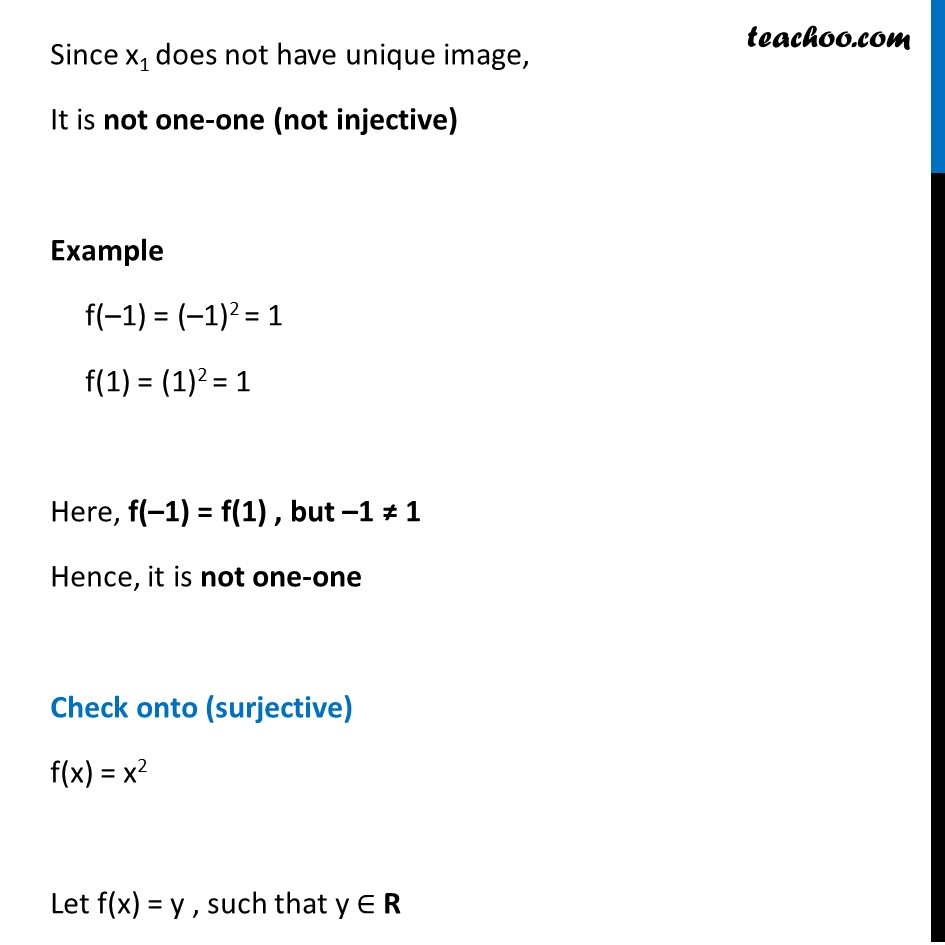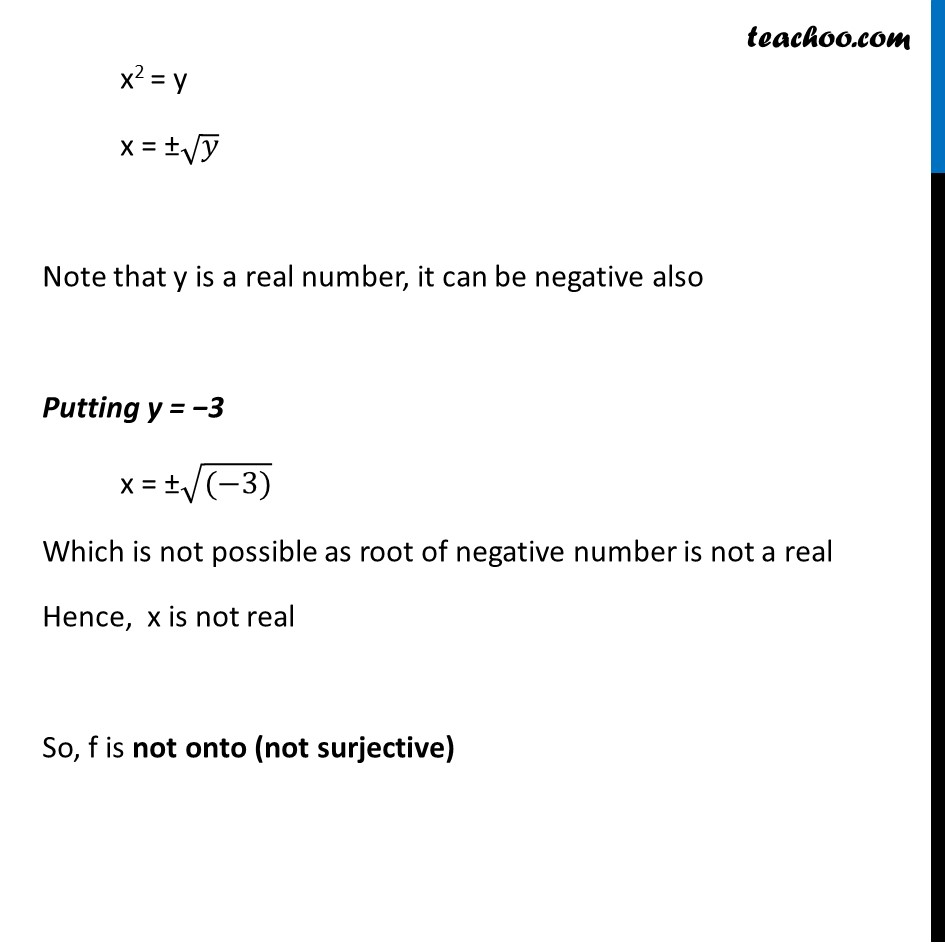Ex 1.2

Chapter 1 Class 12 Relation and Functions
Serial order wiseLearn in your speed, with individual attention - Teachoo Maths 1-on-1 Class

### Transcript

Ex 1.2, 2 Check the injectivity and surjectivity of the following functions: (iii) f: R → R given by f(x) = x2 f(x) = x2 Checking one-one (injective) f (x1) = (x1)2 f (x2) = (x2)2 Putting f (x1) = f (x2) (x1)2 = (x2)2 ∴ x1 = x2 or x1 = –x2 Since x1 does not have unique image, It is not one-one (not injective) Example f(–1) = (–1)2 = 1 f(1) = (1)2 = 1 Here, f(–1) = f(1) , but –1 ≠ 1 Hence, it is not one-one Check onto (surjective) f(x) = x2 Let f(x) = y , such that y ∈ R x2 = y x = ±√𝑦 Note that y is a real number, it can be negative also Putting y = −3 x = ±√((−3)) Which is not possible as root of negative number is not a real Hence, x is not real So, f is not onto (not surjective)